# Half-plane

(diff) ← Older revision | Latest revision (diff) | Newer revision → (diff)
The set of points in a plane situated to one side of a given straight line in that plane. The coordinates of the points of a half-plane satisfy an inequality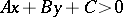, whereare certain constants such thatanddo not vanish simultaneously. If the straight line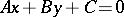itself (the boundary of the half-plane) belongs to the half-plane, the latter is said to be closed. Special half-planes on the complex plane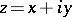are the upper half-plane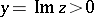, the lower half-plane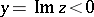, the left half-plane, the right half-plane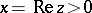, etc. The upper half-plane of the complex-plane can be mapped conformally (cf. Conformal mapping) onto the disc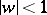by the Möbius transformationwhereis an arbitrary real number and.